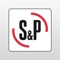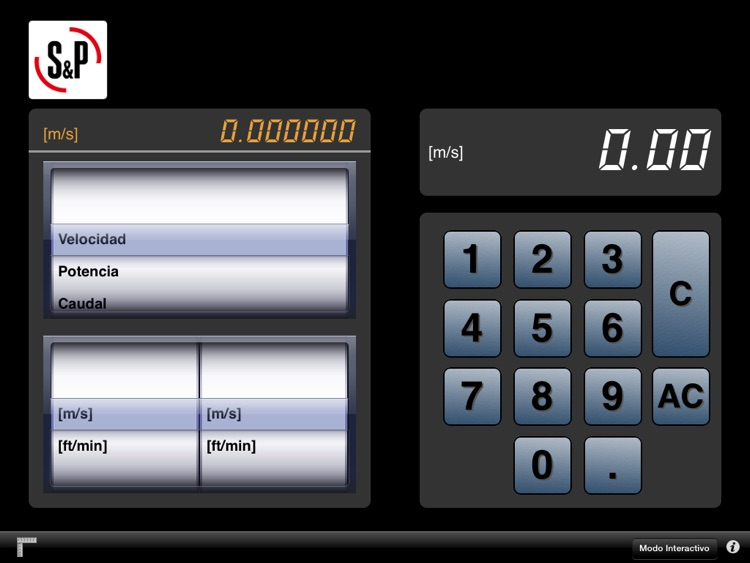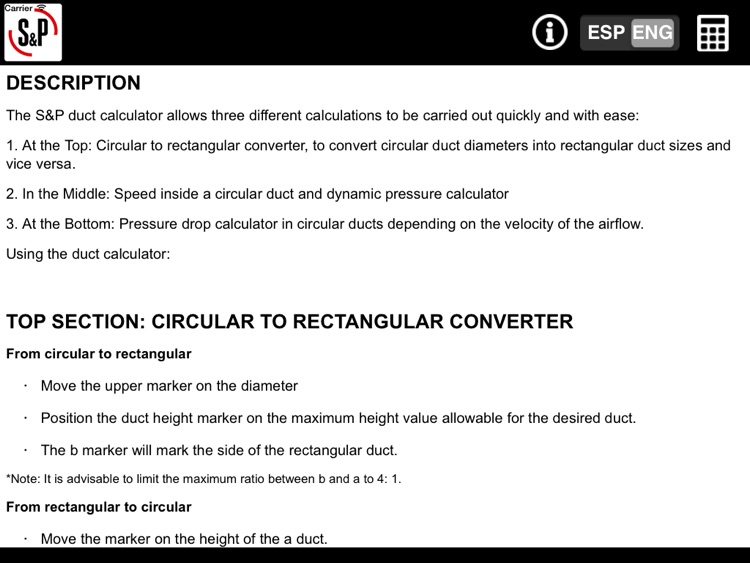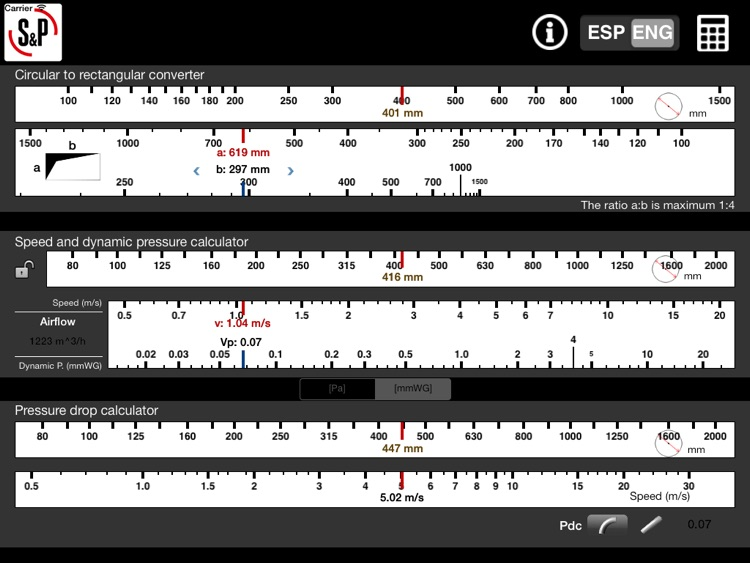## The S&P duct calculator allows three different calculations to be carried out quickly and with ease:# S&P Duct Calculator

by Soler&PalauThe S&P duct calculator allows three different calculations to be carried out quickly and with ease:Version
2.0
Rating
NA
Size
8Mb
Genre
Utilities
Last updated
January 23, 2015
Release date
October 27, 2014

### App Store Description

The S&P duct calculator allows three different calculations to be carried out quickly and with ease:

1) At the Top: conversion of circular duct diameters (mm) Ø into rectangular duct sizes and vice versa.

2) In the Middle: graphic form of Q (m3/h) = V (m/s) · S (m2) · 3600 formula, on the basis of the circular diameter Ø of the duct instead of the S section, in addition to the dynamic pressure calculation.

3) At the Bottom: determination of pressure drop in circular ducts depending on the velocity of the airflow. Using the duct calculator:

1. TOP section: Conversion of circular duct diameter Ø into equivalent rectangular ducts (and vice versa).

1.1 Position the upper cursor on the diameter. Position the duct height cursor on the maximum height value allowable for the desired duct. The b cursor will mark the side of the rectangular duct. It is advisable to limit the maximum ratio between b and a to 4: 1.

1.2 Conversion of rectangular ducts into circular ducts.

Position the cursor on the height of the a duct. Position b on the rectangular side (the numbering moves, not the cursor) and read the corresponding circular diameter Ø on the upper scale.

2. MIDDLE: Determining a third variable, knowing two of them (Q = V · S · 3600)

Knowing the diameter Ø and the air velocity, S and setting a diameter, when moving the velocity cursor, the
corresponding airflow, Q is obtained.
Knowing the diameter and the airflow, it is possible locking the diameter with the help of the lock. Then, we can set the airflow and we will obtain the air velocity, S. Knowing the airflow and the air velocity, S, we can set the desired airflow. Then, placing the velocity cursor on the desired value, we will obtain the corresponding diameter Ø.

2.2 Determining the dynamic pressure.
Position the velocity cursor. Read the dynamic pressure on the bottom scale.

3. BOTTOM: Pressure drop calculation.
Position the cursor on the corresponding diameter. Position the cursor on the velocity of the airflow through the duct. Read the pressure drop per unit length value PCL in the rectangle on the bottom right-hand side. Multiply the obtained value by the total equivalent duct length of the installation to calculate the system pressure drop when installed.